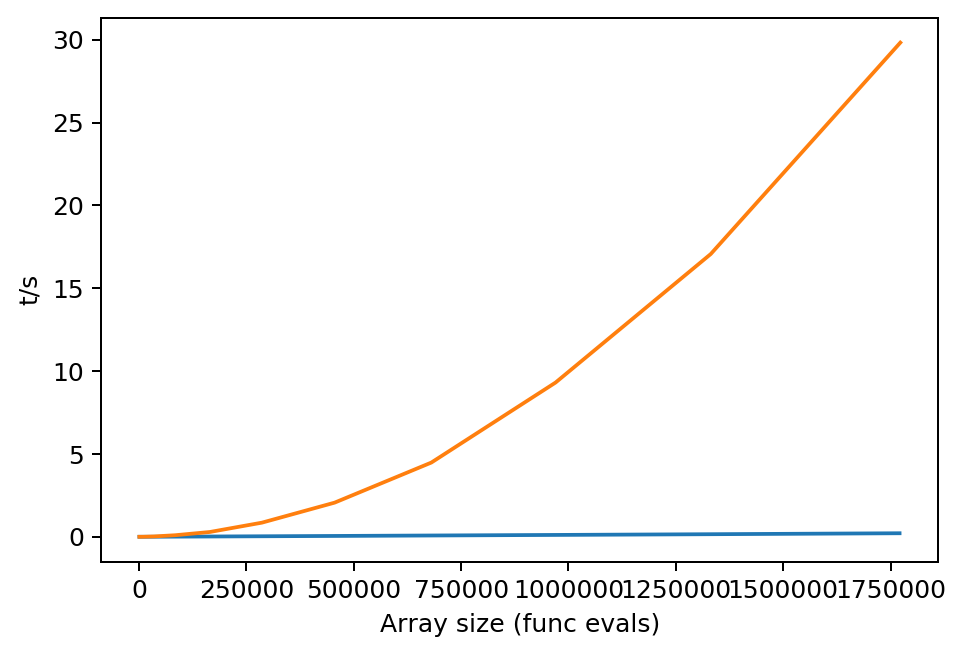## (Moble's) Fast spherical functions with Numba

Tips, discussions, how-tos, libraries...

Paul Hockett
Posts: 22
Joined: Mon May 25, 2020 5:17 pm
Location: Ottawa
Contact:

### (Moble's) Fast spherical functions with Numba

TL;DR; Python Numba acceleration is really fast.

Like faster than Fortan fast.

In my - admittedly still somewhat limited - playing with Python, I've bee very taken with Moble's Spherical Functions library for Wigner D, 3j and spherical harmonics (docs here). This makes use of Numba on the back-end for speed, and is really fast (I haven't compared to "real" codes in C or Fortran, but it's orders of magnitude faster than a basic (looped) Matlab implementation).

Here's how things look for Wigner Ds, as a function of calculation size (J), for Matlab (slow) and Moble's Python implementation (fast). (It's probably worth mentioning that the Matlab code is pretty basic, so could possibly be made somewhat faster than the numbers here, but likely not by orders of magnitude.)As a side-note, the 3j functions in the library are not parallelized over input sets of quantum numbers (the Wigner Ds are, however), but further parallelization here is relatively simple, and fast.

For more general notes on Numba speed, see the great post from Charles Jekel, as quoted above, Comparison of performance: Python NumPy and Numba, MATLAB, and Fortran.